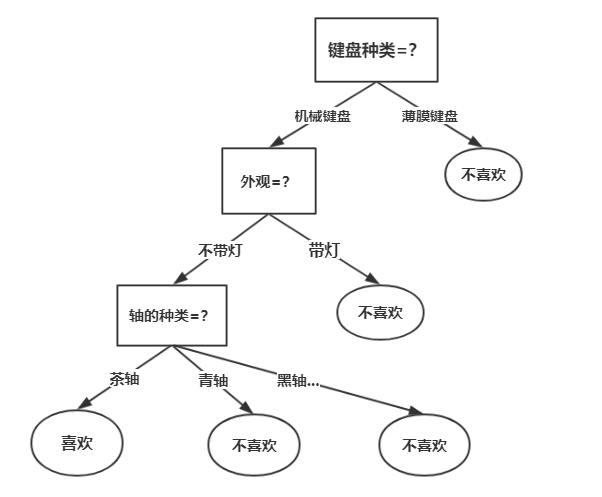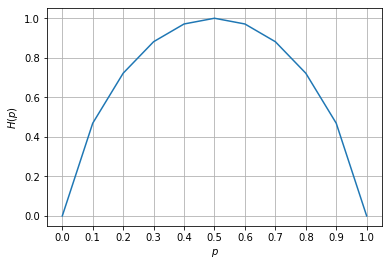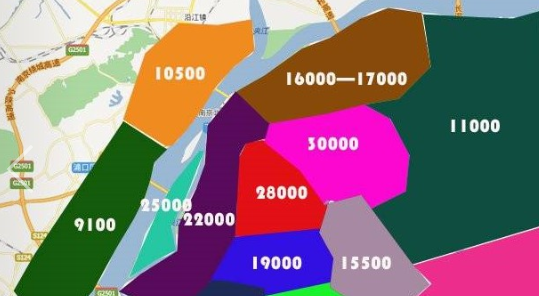1 篇文章 1 订阅

# 决策树模型：

• 内部节点：对应于一个属性测试
• 叶节点：对应于决策结果
• 根节点包含样本全集；
• 每个节点包括的样本集合根据属性测试的结果被划分到子节点中；
• 根节点到每个叶节点的路径对应对应了一个判定测试路径；# 决策树学习算法：

• 特征选择
• 决策树生成
• 决策树剪枝

(下面讨论的都是分类树，回归树会在后面单独再谈)

## 特征选择：

• 信息熵(information entropy)
• 信息增益(information gain)
• 信息增益率(information gain ratio)
• 基尼指数(Gini index)

P(X=xi)=pi,i=1,2,...,n P ( X = x i ) = p i , i = 1 , 2 , . . . , n

$H\left(X\right)=-\sum _{i=1}^{n}{p}_{i}log{p}_{i}$

$P\left(X=1\right)=p,\phantom{\rule{2em}{0ex}}P\left(X=0\right)=1-p,\phantom{\rule{2em}{0ex}}0\le p\le 1$

H(p)=plog2p(1p)log2(1p) H ( p ) = − p l o g 2 p − ( 1 − p ) l o g 2 ( 1 − p )

H(p) H ( p ) $H(p)$的函数图像如下：$H\left(Y|X\right)=\sum _{i=1}^{n}{p}_{i}H\left(Y|X={x}_{i}\right)$

g(D,A)=H(D)H(D|A) g ( D , A ) = H ( D ) − H ( D | A )

• 信息增益的算法:

输入：训练数据集 D D $D$，特征$A$$A$
输出：特征 A A $A$对训练数据集$D$$D$的信息增益 g(D,A) g ( D , A ) $g(D,A)$
(1): ( 1 ) : $(1):$计算数据集 D D $D$的熵$H\left(D\right)$$H(D)$

H(D)=k=1K|Ck||D|log2|Ck||D| H ( D ) = − ∑ k = 1 K | C k | | D | l o g 2 | C k | | D |

(2): ( 2 ) : $(2):$计算特征 A A $A$对训练数据集$D$$D$的条件熵 H(D|A) H ( D | A ) $H(D|A)$
H(D|A)=i=1n|Di||D|H(Di)=i=1n|Di||D|k=1K|Dik||Di|log2|Dik||Di| H ( D | A ) = ∑ i = 1 n | D i | | D | H ( D i ) = − ∑ i = 1 n | D i | | D | ∑ k = 1 K | D i k | | D i | l o g 2 | D i k | | D i |

(3): ( 3 ) : $(3):$计算信息增益
g(D,A)=H(D)H(D|A) g ( D , A ) = H ( D ) − H ( D | A )

|D| | D | $|D|$为样本容量，设有 K K $K$个类${C}_{k},k=1,2,...,K,|{C}_{k}|$$C_{k},k=1,2,...,K,|C_{k}|$为属于类 Ck C k $C_{k}$的样本个数。设特征 A A $A$$n$$n$个不同取值，根据特征 A A $A$的取值将$D$$D$划分为 n n $n$个子集${D}_{1},{D}_{2},...,{D}_{n}$$D_{1},D_{2},...,D_{n}$, Dik D i k $D_{ik}$为子集 Di D i $D_{i}$中属于类 Ck C k ${C_{k}}$的集合。

gR(D,A)=g(D,A)HA(D) g R ( D , A ) = g ( D , A ) H A ( D )

Gini(p)=k=1Kpk(1pk)=1k=1Kp2k G i n i ( p ) = ∑ k = 1 K p k ( 1 − p k ) = 1 − ∑ k = 1 K p k 2

D1= D 1 = $\qquad D_{1}=$ { (x,y)D|A(x)=a ( x , y ) ∈ D | A ( x ) = a $(x,y) \in D|A(x)=a$} ,D2=DD1 , D 2 = D − D 1 $,\quad D_{2}=D-D_{1}$

Gini(D,A=a)=|D1||D|Gini(D1)+|D2||D|Gini(D2) G i n i ( D , A = a ) = | D 1 | | D | G i n i ( D 1 ) + | D 2 | | D | G i n i ( D 2 )

## 决策树生成

ID3 I D 3 $ID3$信息增益
C4.5 C 4.5 $C4.5$信息增益率
CART C A R T $CART$基尼指数

## ID3: I D 3 : $ID3:$

ID3 I D 3 $ID3$算法的核心是在决策树各个节点上根据信息增益来选择进行划分的特征，然后递归地构建决策树。

1. 从根节点开始，对节点计算所有可能的特征的信息增益，选择信息增益值最大的特征作为节点的划分特征；
2. 由该特征的不同取值建立子节点；
3. 再对子节点递归地调用以上方法，构建决策树；
4. 到所有特征的信息增益都很小或者没有特征可以选择为止，得到最终的决策树

ID3 I D 3 $ID3$的局限：

1. 没有剪枝
2. 采用信息增益作为选择最优划分特征的标准，然而信息增益会偏向那些取值较多的特征(这也是 C4.5 C 4.5 $C4.5$采用信息增益率的原因)

## C4.5: C 4.5 : $C4.5:$

C4.5 C 4.5 $C4.5$ ID3 I D 3 $ID3$相似，但对 ID3 I D 3 $ID3$进行了改进，在这里不再详细描述 C4.5 C 4.5 $C4.5$的实现，就讲一下有哪些基于 ID3 I D 3 $ID3$的改进：

1. 用信息增益率来选择划分特征，克服了用信息增益选择的不足
2. 在构造树的过程中进行剪枝
3. 对连续值与缺失值进行处理

## 为什么 C4.5 C 4.5 $C4.5$要用信息增益率代替信息增益？为什么信息增益会偏向多取值特征？

${g}_{R}\left(D,A\right)=\frac{g\left(D,A\right)}{{H}_{A}\left(D\right)}$

(我的理解如果有什么错误的地方的话，希望大家能够指正，不胜感激XD)

## CART: C A R T : $CART:$

CART(classificationandregressiontree), C A R T ( c l a s s i f i c a t i o n a n d r e g r e s s i o n t r e e ) , $CART(classification and regression tree),$分类回归树算法，既可用于分类也可用于回归，在这一部分我们先主要将其分类树的生成。区别于 ID3 I D 3 $ID3$ C4.5 C 4.5 $C4.5$, CART C A R T $CART$假设决策树是二叉树，内部节点特征的取值为“是”和“否”，左分支为取值为“是”的分支，右分支为取值为”否“的分支。这样的决策树等价于递归地二分每个特征，将输入空间(即特征空间)划分为有限个单元。 CART C A R T $CART$的分类树用基尼指数来选择最优特征的最优划分点，具体过程如下

1. 从根节点开始，对节点计算现有特征的基尼指数，对每一个特征，例如 A A $A$，再对其每个可能的取值如$a$$a$,根据样本点对 A=a A = a $A=a$的结果的”是“与”否“划分为两个部分，利用 Gini(D,A=a)=|D1||D|Gini(D1)+|D2||D|Gini(D2) G i n i ( D , A = a ) = | D 1 | | D | G i n i ( D 1 ) + | D 2 | | D | G i n i ( D 2 ) $Gini(D,A=a)=\frac{|D_{1}|}{|D|}Gini(D_{1})+\frac{|D_{2}|}{|D|}Gini(D_{2})$进行计算；
2. 在所有可能的特征 A A $A$以及该特征所有的可能取值$a$$a$，选择基尼指数最小的特征及其对应的取值作为最优特征和最优切分点。然后根据最优特征和最优切分点，将本节点的数据集二分，生成两个子节点
3. 对两个字节点递归地调用上述步骤，直至节点中的样本个数小于阈值，或者样本集的基尼指数小于阈值，或者没有更多特征后停止；
4. 生成 CART C A R T $CART$分类树；

## 回归树：

$\sum _{{x}_{i}\in {R}_{m}}\left({y}_{i}-f\left({x}_{i}\right){\right)}^{2}$

f(xi)=cm=ave(yi|xiRm) f ( x i ) = c m = a v e ( y i | x i ∈ R m )

(将输入空间划分为 M M $M$个单元${R}_{1},{R}_{2},...,{R}_{m}$$R_{1},R_{2},...,R_{m}$)

minj,s[minc1xiR1(j,s)(yic1)2+minc2xiR2(j,s)(yic2)2] m i n j , s [ m i n c 1 ∑ x i ∈ R 1 ( j , s ) ( y i − c 1 ) 2 + m i n c 2 ∑ x i ∈ R 2 ( j , s ) ( y i − c 2 ) 2 ]

1. 选择最优的切分变量 j j $j$和最优的切分点$s$$s$，求解
minj,s[minc1xiR1(j,s)(yic1)2+minc2xiR2(j,s)(yic2)2] m i n j , s [ m i n c 1 ∑ x i ∈ R 1 ( j , s ) ( y i − c 1 ) 2 + m i n c 2 ∑ x i ∈ R 2 ( j , s ) ( y i − c 2 ) 2 ]

遍历所有特征，对固定的特征扫描所有取值，找到使上式达到最小值的对 (j,s) ( j , s ) $(j,s)$.
2. 用选定的对 (j,s) ( j , s ) $(j,s)$划分区域，并确定该区域的预测值；
3. 继续对两个字区域调用上述步骤，直至满足停止条件；
4. 生成回归树；(图片来源于网络，如有侵权会马上删除：)

## 参考文献

 李航. 统计学习方法[M]. 北京：清华大学出版社，2012

07-02
09-1723万+08-20
02-09
03-191万+
08-203万+
09-102万+
05-11702
01-28149
07-08383
08-228049
03-263990
08-295506
05-2469
12-05395
04-11326
04-0719
06-226174
08-212259点击重新获取扫码支付余额充值